## Machine learning in Perl, Part2: a calculator, handwritten digits and roboshakespeare.

Hello all,
In my first blog post I've announced AI::MXNet, Perl interface to the MXNet machine learning library.
The eight weeks that passed after that were quite fruitful, I've ported whole python's test suite, fixed multiple bugs, added docs, examples, high level RNN interface, Perl API docs has been added to the official MXNet website.
This time I'd like to review in detail three examples from the examples directory.
First one is a simple calculator, a fully connected net that is structured to learn four basic arithmetic operators: addition, subtraction, multiplication and division.
Second example is a comparison of two different approaches to the task of classification of handwritten digits, and the third one is an example of LSTM RNN network trained to generate Shakespeare like text.

Here is the image (generated by Graphviz) of the calculator network.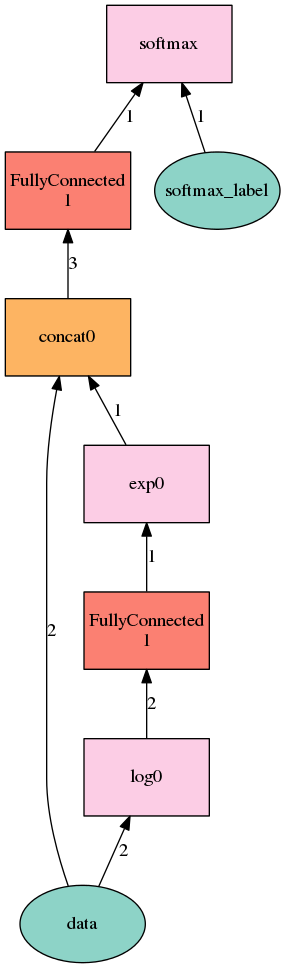The data input is two numbers, that are being routed via two paths; first path is turning the input values into natural logarithms and feeds these into one neuron sized fully connected layer.
This neuron has two weights (floats) that each of logarithm values are multiplied by and then
summed, it also has a bias (another float) that is added to the sum. That value is the output
of this fully connected layer.
Then we apply an exponent function to the output, essentially getting either the product or the quotient of the two input values with appropriate weight and bias values that network is supposed to learn from the examples.
Second path is taking the original unadulterated input values along with output of the first fully connected layer and feeds these three values into second one neuron sized fully connected layer.
This neuron has three weights and a bias and its output is the final answer.
The goal of the network is to learn to ignore one of the paths and use another for the specific function.
Here is one way how these weights can be arranged:

 First layer Second layer w1 w2 bias w1 w2 w3 bias Addition 0 0 0 1 1 0 0 Subtraction 0 0 0 1 -1 0 0 Multiplication 1 1 0 0 0 1 0 Division 1 -1 0 0 0 1 0

Which corresponds to following functions:
``````
x+y = (exp(0 * log(x) + 0 * log(y)) + 0) * 0 + 1 * x + 1 * y + 0
x-y = (exp(0 * log(x) + 0 * log(y)) + 0) * 0 + 1 * x + -1 * y + 0
x*y = (exp(1 * log(x) + 1 * log(y)) + 0) * 1 + 0 * x + 0 * y + 0
x/y = (exp(1 * log(x) + -1 * log(y)) + 0) * 1 + 0 * x + 0 * y + 0
``````

That's all there is to it, seems pretty simple. However even this simple network hides many interesting lessons under the hood.

Let's put it under the microscope. Here is the script using AI::MXNet that implements the network.

``````
#!/usr/bin/perl
use strict;
use warnings;
use AI::MXNet ('mx');

## preparing the samples
## to train our network
sub samples {
my(\$batch_size, \$func) = @_;
# get samples
my \$n = 16384;
## creates a pdl with \$n rows and two columns with random
## floats in the range between 0 and 1
my \$data = PDL->random(2, \$n);
## creates the pdl with \$n rows and one column with labels
## labels are floats that either sum or product, etc of
## two random values in each corresponding row of the data pdl
my \$label = \$func->(\$data->slice('0,:'), \$data->slice('1,:'));
# partition into train/eval sets
my \$edge = int(\$n / 8);
my \$validation_data = \$data->slice(":,0:@{[ \$edge - 1 ]}");
my \$validation_label = \$label->slice(":,0:@{[ \$edge - 1 ]}");
my \$train_data = \$data->slice(":,\$edge:");
my \$train_label = \$label->slice(":,\$edge:");
# build iterators around the sets
return(mx->io->NDArrayIter(
batch_size => \$batch_size,
data => \$train_data,
label => \$train_label,
), mx->io->NDArrayIter(
batch_size => \$batch_size,
data => \$validation_data,
label => \$validation_label,
));
}
## the network model
sub nn_fc {
my \$data = mx->sym->Variable('data');
my \$ln = mx->sym->exp(mx->sym->FullyConnected(
data => mx->sym->log(\$data),
num_hidden => 1,
));
my \$wide = mx->sym->Concat(\$data, \$ln);
my \$fc = mx->sym->FullyConnected(
\$wide,
num_hidden => 1
);
return mx->sym->MAERegressionOutput(data => \$fc,
name => 'softmax');
}

sub learn_function {
my(%args) = @_;
my \$func = \$args{func};
my \$batch_size = \$args{batch_size}//128;
my(\$train_iter, \$eval_iter) = samples(\$batch_size, \$func);
my \$sym = nn_fc();

## call as ./calculator.pl 1 to just print model and exit
if(\$ARGV) {
my @dsz = @{\$train_iter->data->->shape};
my @lsz = @{\$train_iter->label->->shape};
my \$shape = {
data          => [ \$batch_size, splice @dsz,  1 ],
softmax_label => [ \$batch_size, splice @lsz, 1 ],
};
print mx->viz->plot_network(\$sym,
shape => \$shape)->graph->as_png;
exit;
}

my \$model = mx->mod->Module(
symbol => \$sym,
context => mx->cpu(),
);
\$model->fit(\$train_iter,
eval_data => \$eval_iter,
optimizer_params => {
learning_rate => \$args{lr}//0.01,
#lr_scheduler  => AI::MXNet::FactorScheduler->new(
#    step => 100,
#    factor => 0.99
#)
},
eval_metric => 'mse',
num_epoch => \$args{epoch}//25,
);

# refit the model for calling on 1 sample at a time
my \$iter = mx->io->NDArrayIter(
batch_size => 1,
data => PDL->pdl([[ 0, 0 ]]),
label => PDL->pdl([[ 0 ]]),
);
\$model->reshape(
data_shapes => \$iter->provide_data,
label_shapes => \$iter->provide_label,
);

# wrap a helper around making predictions
my (\$arg_params) = \$model->get_params;
for my \$k (sort keys %\$arg_params)
{
print "\$k -> ". \$arg_params->{\$k}->aspdl."\n";
}
return sub {
my(\$n, \$m) = @_;
return \$model->predict(mx->io->NDArrayIter(
batch_size => 1,
data => PDL->new([[ \$n, \$m ]]),
))->aspdl->list;
};
}

my \$add = learn_function(func => sub {
my(\$n, \$m) = @_;
return \$n + \$m;
});
my \$sub = learn_function(func => sub {
my(\$n, \$m) = @_;
return \$n - \$m;
},
# batch_size => 50, epoch => 40
);
my \$mul = learn_function(func => sub {
my(\$n, \$m) = @_;
return \$n * \$m;
},
# batch_size => 50, epoch => 40
);
my \$div = learn_function(func => sub {
my(\$n, \$m) = @_;
return \$n / \$m;
},
# batch_size => 10, epoch => 80
);
print "12345 + 54321 ≈ ", \$add->(12345, 54321), "\n";
print "188 - 88 ≈ ", \$sub->(188, 88), "\n";
print "250 * 2 ≈ ", \$mul->(250, 2), "\n";
print "250 / 2 ≈ ", \$div->(250, 2), "\n";
```
```
Training the network with the default parameters produces good results for an addition, subtraction and multiplication, but does not work too well for the division, the loss just oscillates around, never converging on the minimum.
This is usually a sign of learning rate being too large leading to the constant overshooting.
``````
...
Epoch Train-mse=365.166418
Epoch Time cost=0.110
Epoch Validation-mse=220.828789
Epoch Train-mse=265.768834
Epoch Time cost=0.127
Epoch Validation-mse=303.636722
Epoch Train-mse=197.760114
Epoch Time cost=0.106
Epoch Validation-mse=358.861350
Epoch Train-mse=456.315173
Epoch Time cost=0.391
Epoch Validation-mse=1635.785243
Epoch Train-mse=32425.546294
Epoch Time cost=0.123
Epoch Validation-mse=70.104976

12345 + 54321 ≈ 67207.34375
188 - 88 ≈ 100.019081115723
250 * 2 ≈ 506.744720458984
250 / 2 ≈ 167.313049316406
```
```
In AI::MXNet it's easy to anneal the learning rate, we only have to define the lr_scheduler parameter for our optimizer; optimizer is the subsystem that updates the network weights (on the first run we had that code commented out).
As well, to give the network a bit more granular and unique input, it is desirable to decrease the batch size, say to 10 samples and increase the number of training epochs to about of 80.
And presto, we have working division network.
``````
...
Epoch Train-mse=0.000000
Epoch Time cost=1.458
Epoch Validation-mse=0.000005
Update: Change learning rate to 1.13416e-07
Update: Change learning rate to 1.12282e-07
Update: Change learning rate to 1.11159e-07
....
Update: Change learning rate to 1.00530e-07
Update: Change learning rate to 9.95252e-08
Update: Change learning rate to 9.85299e-08
Epoch Train-mse=0.000000
Epoch Time cost=1.427
Epoch Validation-mse=0.000001

12345 + 54321 ≈ 66778.6328125
188 - 88 ≈ 99.8619995117188
250 * 2 ≈ 497.708892822266
250 / 2 ≈ 125.000076293945
```
```

While playing with the batch size I discovered that setting the batch size to 10 completely overfits the addition network and it becomes unable to properly function outside of the range of direct samples.

This exercise showed us that choosing correct hyper parameters is very important for a determining correct network structure for some specific task.
The 'calculator' network is unambiguously works for sub, div and mul, but the 'add' function can possibly be fit via both logic paths, log/exp and simple addition, so when given the chance the network happily overfits and loses its generic abilities.
This is something that every ML engineer has to be aware of.
Now, it would be interesting to see what kinds of solutions the network has discovered. There's the way to look under the hood and for this network is especially easy because it's so small.
The AI::MXNet is seamlessly glued with PDL and it's very easy to transfer its internal structures (AI::MXNet::NDArray) to the PDL objects to be printed.
Here is how we'll do it:
``````
my (\$arg_params) = \$model->get_params;
for my \$k (sort keys %\$arg_params)
{
print "\$k -> ". \$arg_params->{\$k}->aspdl."\n";
}
```
```
And here are the results from one of the runs:
``````
fullyconnected0_bias -> [-0.827079]
fullyconnected0_weight ->
[
[0.653499  0.85165]
]

fullyconnected1_bias -> [-0.000376125]
fullyconnected1_weight ->
[
[     1.00095       1.0007 -6.34936e-05]
]

# subtraction
fullyconnected2_bias -> [0.0330982]
fullyconnected2_weight ->
[
[0.00111382    1.00181]
]

fullyconnected3_bias -> [6.35026e-05]
fullyconnected3_weight ->
[
[  1.00076 -0.761943  -0.23057]
]

# multiplication
fullyconnected4_bias -> [0.677846]
fullyconnected4_weight ->
[
[0.999995 0.998743]
]

fullyconnected5_bias -> [-0.000313076]
fullyconnected5_weight ->
[
[-0.000252387 -0.000403451     0.507238]
]

# division
fullyconnected6_bias -> [0.410057]
fullyconnected6_weight ->
[
[  1.00001 -0.999997]
]

fullyconnected7_bias -> [6.66899e-07]
fullyconnected7_weight ->
[
[-2.39469e-05  6.82368e-06     0.663621]
]

12345 + 54321 ≈ 66574.765625
188 - 88 ≈ 99.8238220214844
250 * 2 ≈ 499.02294921875
250 / 2 ≈ 124.999740600586
```
```
Unsurprisingly, the network driven by randomness is much more inventive than the simplest variant I thought up above.

Next example.
The MNIST problem is a 'Hello World!' task for neural networks, it's not that hard, but in the same time is not easy to solve using completely imperative hand coded approach due to many exceptions that inherently exist in the imperfect hand written digits.
Here is the gist of it. Few decades ago, a few hundred or so students and workers of American census produced 70000 hand written digit samples.
These then were digitized and converted into 28x28 gray images.
The example below compares the performance of fully connected and the convolutional network.

``````
#!/usr/bin/perl
use strict;
use warnings;
# derived from http://mxnet.io/tutorials/python/mnist.html
use LWP::UserAgent ();
use PDL ();
use AI::MXNet ('mx');

my \$ua = LWP::UserAgent->new();

my \$fname = (split /\//, \$url)[-1];
\$ua->get(\$url, ':content_file' => \$fname);
}
return \$fname;
}

my(\$label_url, \$image_url) = @_;
my(\$magic, \$num, \$rows, \$cols);

(\$magic, \$num) = unpack 'N2', \$buf;
my \$label = PDL->new();
\$label->set_datatype(\$PDL::Types::PDL_B);
\$label->setdims([ \$num ]);
\$label->upd_data();

(\$magic, \$num, \$rows, \$cols) = unpack 'N4', \$buf;
my \$image = PDL->new();
\$image->set_datatype(\$PDL::Types::PDL_B);
\$image->setdims([ \$rows, \$cols, \$num ]);
read \$fimg, \${\$image->get_dataref}, \$num * \$rows * \$cols;
\$image->upd_data();
return(\$label, \$image);
}

my \$path='http://yann.lecun.com/exdb/mnist/';
"\${path}train-labels-idx1-ubyte.gz", "\${path}train-images-idx3-ubyte.gz");
"\${path}t10k-labels-idx1-ubyte.gz", "\${path}t10k-images-idx3-ubyte.gz");

sub to4d {
my(\$img) = @_;
return \$img->reshape(28, 28, 1, (\$img->dims))->float / 255;
}

my \$batch_size = 100;
my \$train_iter = mx->io->NDArrayIter(
data => to4d(\$train_img),
label => \$train_lbl,
batch_size => \$batch_size,
shuffle => 1,
);
my \$val_iter = mx->io->NDArrayIter(
data => to4d(\$val_img),
label => \$val_lbl,
batch_size => \$batch_size,
);

# Create a place holder variable for the input data
my \$data = mx->sym->Variable('data');

sub nn_fc {
# Epoch Train-accuracy=0.978889
# Epoch Time cost=145.437
# Epoch Validation-accuracy=0.964600
my(\$data) = @_;

# Flatten the data from 4-D shape (batch_size, num_channel, width, height)
# into 2-D (batch_size, num_channel*width*height)
\$data = mx->sym->Flatten(data => \$data);

# The first fully-connected layer
#    my \$fc1  = mx->sym->FullyConnected(data => \$data, name => 'fc1', num_hidden => 128);
#    # Apply relu to the output of the first fully-connnected layer
#    my \$act1 = mx->sym->Activation(data => \$fc1, name => 'relu1', act_type => "relu");

# The second fully-connected layer and the according activation function
my \$fc2  = mx->sym->FullyConnected(data => \$data, name => 'fc2', num_hidden => 64);
my \$act2 = mx->sym->Activation(data => \$fc2, name => 'relu2', act_type => "relu");

# The thrid fully-connected layer, note that the hidden size should be 10, which is the number of unique digits
my \$fc3  = mx->sym->FullyConnected(data => \$act2, name => 'fc3', num_hidden => 10);
# The softmax and loss layer
my \$mlp  = mx->sym->SoftmaxOutput(data => \$fc3, name => 'softmax');
return \$mlp;
}

sub nn_conv {
my(\$data) = @_;
# Epoch Batch       Speed: 1625.07 samples/sec      Train-accuracy=0.992090
# Epoch Batch       Speed: 1630.12 samples/sec      Train-accuracy=0.992850
# Epoch Train-accuracy=0.991357
# Epoch Time cost=36.817
# Epoch Validation-accuracy=0.988100

my \$conv1= mx->symbol->Convolution(data => \$data, name => 'conv1', num_filter => 20, kernel => [5,5], stride => [2,2]);
my \$bn1  = mx->symbol->BatchNorm(data => \$conv1, name => "bn1");
my \$act1 = mx->symbol->Activation(data => \$bn1, name => 'relu1', act_type => "relu");
my \$mp1  = mx->symbol->Pooling(data => \$act1, name => 'mp1', kernel => [2,2], stride =>[1,1], pool_type=>'max');

my \$conv2= mx->symbol->Convolution(data => \$mp1, name => 'conv2', num_filter => 50, kernel=>[3,3], stride=>[2,2]);
my \$bn2  = mx->symbol->BatchNorm(data => \$conv2, name=>"bn2");
my \$act2 = mx->symbol->Activation(data => \$bn2, name=>'relu2', act_type=>"relu");
my \$mp2  = mx->symbol->Pooling(data => \$act2, name => 'mp2', kernel=>[2,2], stride=>[1,1], pool_type=>'max');

my \$fl   = mx->symbol->Flatten(data => \$mp2, name=>"flatten");
my \$fc1  = mx->symbol->FullyConnected(data => \$fl,  name=>"fc1", num_hidden=>100);
my \$act3 = mx->symbol->Activation(data => \$fc1, name=>'relu3', act_type=>"relu");
my \$fc2  = mx->symbol->FullyConnected(data => \$act3, name=>'fc2', num_hidden=>30);
my \$act4 = mx->symbol->Activation(data => \$fc2, name=>'relu4', act_type=>"relu");
my \$fc3  = mx->symbol->FullyConnected(data => \$act4, name=>'fc3', num_hidden=>10);
my \$softmax = mx->symbol->SoftmaxOutput(data => \$fc3, name => 'softmax');
return \$softmax;
}

my \$mlp = \$ARGV ? nn_conv(\$data) : nn_fc(\$data);
#We visualize the network structure with output size (the batch_size is ignored.)
#my \$shape = { data => [ \$batch_size, 1, 28, 28 ] };
#show_network(mx->viz->plot_network(\$mlp, shape => \$shape));

my \$model = mx->mod->Module(
symbol => \$mlp,       # network structure
);
\$model->fit(
\$train_iter,       # training data
num_epoch => 10,      # number of data passes for training
eval_data => \$val_iter, # validation data
batch_end_callback => mx->callback->Speedometer(\$batch_size, 200), # output progress for each 200 data batches
);
```
```

A fully connected network tops out at 97% classification accuracy and the convolutional network gets a bit above 99%.
Below are images of both models.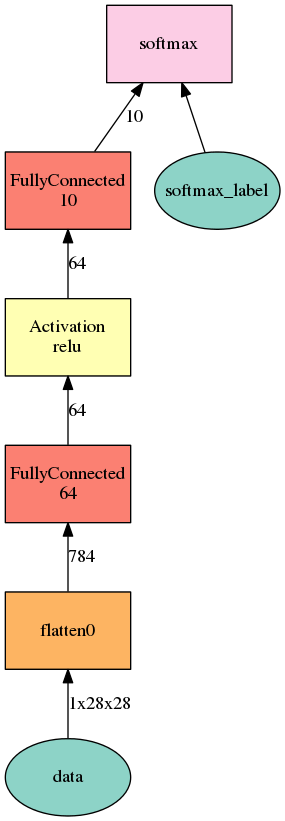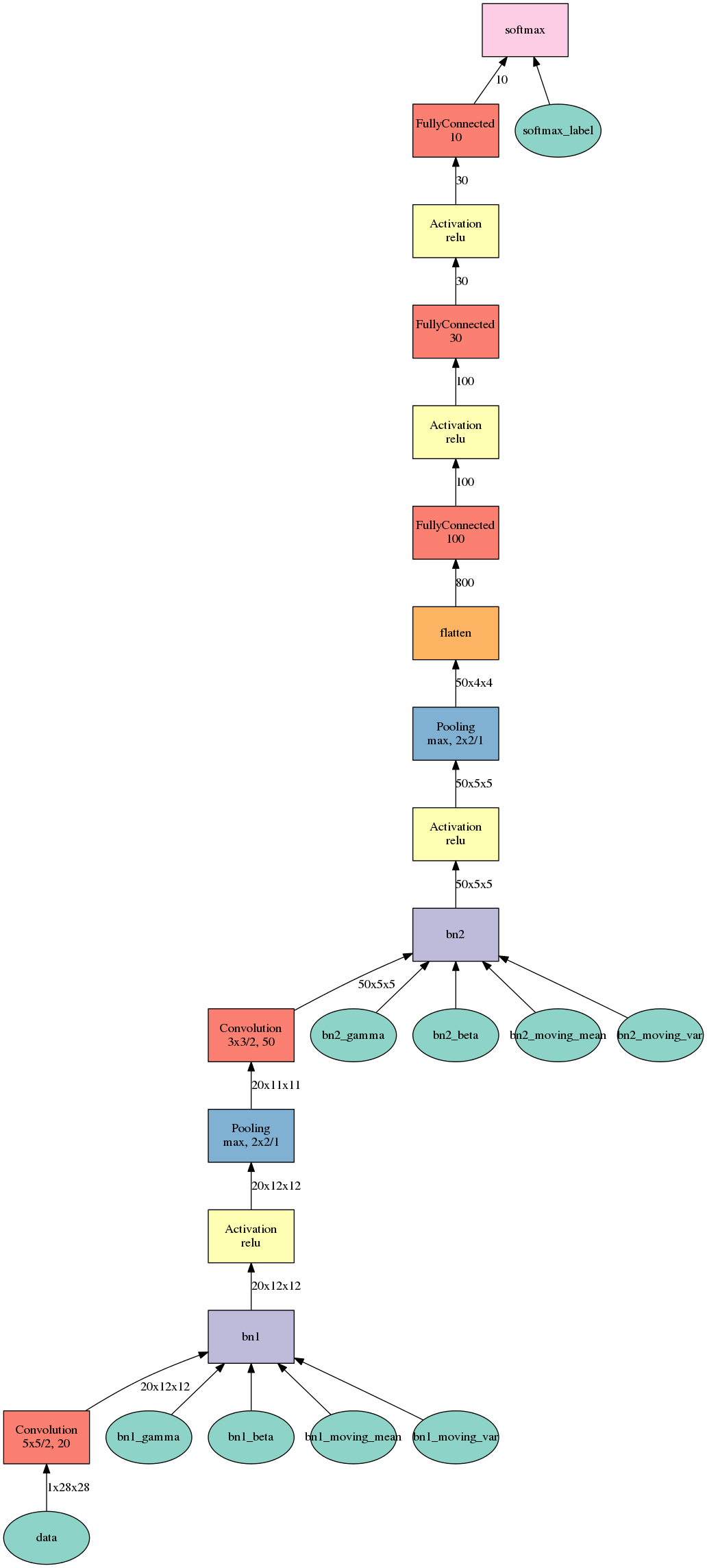This is too simple of an example to show you full power of the convolutional networks, check out this youtube video for real cool stuff!
The convolutional networks are pretty sensitive to the kernel size, number of feature filters and especially to the pool stride value; to get best results I needed to play with the parameters.
That's something that is important to keep in mind when constructing the convolutional networks.

Last example.
The fully connected and convolutional networks have something in common. They share the same weakness.
They accept inputs and deliver results starting from scratch, ignoring all previous inputs. This works well when there's a need to classify images or other independent input but simply does not work for a structured sentence of inputs, a most basic example being a sequence of characters.
There's another type of neural net that can master such tasks, so called recurrent neural networks.
AI::MXNet supports three most popular models: plain vanilla RNN, Gated Recurrent Unit (GRU) and Long Short-Term Memory (LSTM) recurrent neural networks.
For this example I use LSTM and to make it even more powerful, a bidirectional net structure and train my model on GPU.
Training on GPU is something MXNet is especially good at.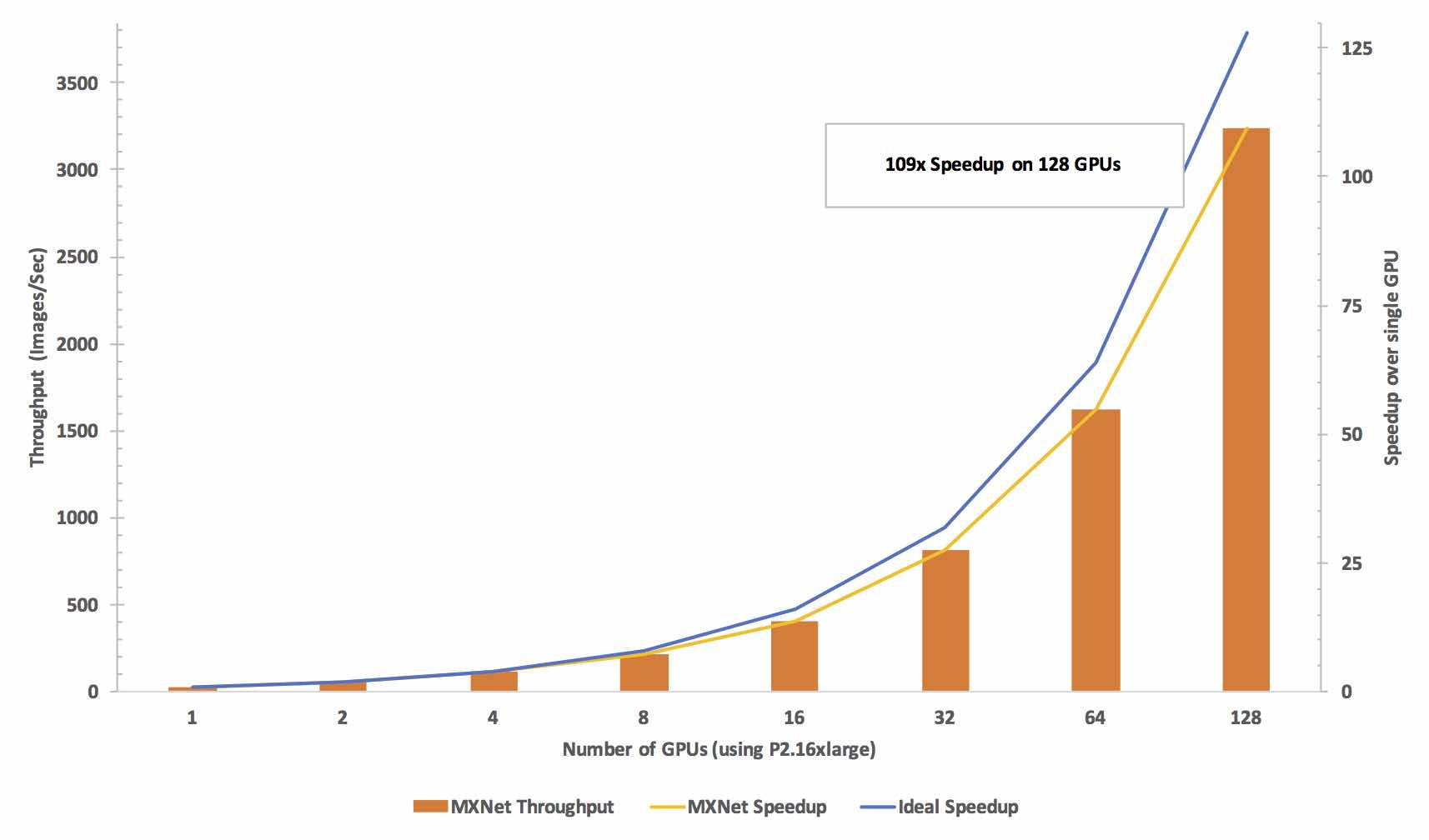I also use annealing of learning rate and relatively small batch size to ease my network into the convergence.
AI::MXNet makes it easy to monitor the network performance via wide set of predefined metrics, while allowing you to create custom ones for the special cases.
For this particular case a stock Perplexity metric works sufficiently good.
Below are the script and the image of the model (extremely simplified, the sequence length is just two chars, I used 60 char long RNN for the training).

``````
#!/usr/bin/perl
use strict;
use warnings;
use PDL;
use Math::Random::Discrete;
use AI::MXNet qw(mx);
use AI::MXNet::Function::Parameters;
use Getopt::Long qw(HelpMessage);

GetOptions(
'num-layers=i'   => \(my \$num_layers   = 2       ),
'num-hidden=i'   => \(my \$num_hidden   = 256     ),
'num-embed=i'    => \(my \$num_embed    = 10      ),
'num-seq=i'      => \(my \$seq_size     = 60      ),
'gpus=s'         => \(my \$gpus                   ),
'kv-store=s'     => \(my \$kv_store     = 'device'),
'num-epoch=i'    => \(my \$num_epoch    = 25      ),
'lr=f'           => \(my \$lr           = 0.001    ),
'optimizer=s'    => \(my \$optimizer    = 'adam'   ),
'mom=f'          => \(my \$mom          = 0       ),
'wd=f'           => \(my \$wd           = 0.00001 ),
'batch-size=i'   => \(my \$batch_size   = 32      ),
'disp-batches=i' => \(my \$disp_batches = 50      ),
'chkp-prefix=s'  => \(my \$chkp_prefix  = 'lstm_' ),
'cell-mode=s'    => \(my \$cell_mode    = 'LSTM'  ),
'sample-size=i'  => \(my \$sample_size  = 10000   ),
'chkp-epoch=i'   => \(my \$chkp_epoch   = 1       ),
'bidirectional=i'=> \(my \$bidirectional= 0       ),
'help'           => sub { HelpMessage(0) },
) or HelpMessage(1);

char_lstm.pl - Example of training char LSTM RNN on tiny shakespeare using high level RNN interface
with optional inferred sampling (RNN generates Shakespeare like text)

--num-layers     number of stacked RNN layers, default=2
--num-hidden     hidden layer size, default=256
--num-embed      embed size, default=10
--num-seq        sequence size, default=60
--gpus           list of gpus to run, e.g. 0 or 0,2,5. empty means using cpu.
Increase batch size when using multiple gpus for best performance.
--kv-store       key-value store type, default='device'
--num-epochs     max num of epochs, default=25
--lr             initial learning rate, default=0.01
--mom            momentum for sgd, default=0.0
--wd             weight decay for sgd, default=0.00001
--batch-size     the batch size type, default=32
--bidirectional  use bidirectional cell, default false (0)
--disp-batches   show progress for every n batches, default=50
--chkp-prefix    prefix for checkpoint files, default='lstm_'
--cell-mode      RNN cell mode (LSTM, GRU, RNN, default=LSTM)
--sample-size    a size of inferred sample text (default=10000) after each epoch
--chkp-epoch     save checkpoint after this many epoch, default=1 (saving every checkpoint)

=cut

package AI::MXNet::RNN::IO::ASCIIIterator;
use Mouse;
extends AI::MXNet::DataIter;
has 'data'          => (is => 'ro',  isa => 'PDL',   required => 1);
has 'seq_size'      => (is => 'ro',  isa => 'Int',   required => 1);
has '+batch_size'   => (is => 'ro',  isa => 'Int',   required => 1);
has 'data_name'     => (is => 'ro',  isa => 'Str',   default => 'data');
has 'label_name'    => (is => 'ro',  isa => 'Str',   default => 'softmax_label');
has 'dtype'         => (is => 'ro',  isa => 'Dtype', default => 'float32');
has [qw/nd counter seq_counter vocab_size
data_size provide_data provide_label idx/] => (is => 'rw', init_arg => undef);

sub BUILD
{
my \$self = shift;
\$self->data_size(\$self->data->nelem);
my \$segments = int((\$self->data_size-\$self->seq_size)/(\$self->batch_size*\$self->seq_size));
\$self->idx([0..\$segments-1]);
\$self->vocab_size(\$self->data->uniq->shape->at(0));
\$self->counter(0);
\$self->seq_counter(0);
\$self->nd(mx->nd->array(\$self->data, dtype => \$self->dtype));
my \$shape = [\$self->batch_size, \$self->seq_size];
\$self->provide_data([
name  => \$self->data_name,
shape => \$shape,
dtype => \$self->dtype
)
]);
\$self->provide_label([
name  => \$self->label_name,
shape => \$shape,
dtype => \$self->dtype
)
]);
\$self->reset;
}

method reset()
{
\$self->counter(0);
@{ \$self->idx } = List::Util::shuffle(@{ \$self->idx });
}

method next()
{
return undef if \$self->counter == @{\$self->idx};
my \$offset = \$self->idx->[\$self->counter]*\$self->batch_size*\$self->seq_size + \$self->seq_counter;
my \$data = \$self->nd->slice(
[\$offset, \$offset + \$self->batch_size*\$self->seq_size-1]
)->reshape([\$self->batch_size, \$self->seq_size]);
my \$label = \$self->nd->slice(
[\$offset + 1 , \$offset + \$self->batch_size*\$self->seq_size]
)->reshape([\$self->batch_size, \$self->seq_size]);
\$self->seq_counter(\$self->seq_counter + 1);
if(\$self->seq_counter == \$seq_size - 1)
{
\$self->counter(\$self->counter + 1);
\$self->seq_counter(0);
}
return AI::MXNet::DataBatch->new(
data          => [\$data],
label         => [\$label],
provide_data  => [
name  => \$self->data_name,
shape => \$data->shape,
dtype => \$self->dtype
)
],
provide_label => [
name  => \$self->label_name,
shape => \$label->shape,
dtype => \$self->dtype
)
],
);
}

package main;
my \$file = "data/input.txt";
open(F, \$file) or die "can't open \$file: \$!";
my \$fdata;
{ local(\$/) = undef; \$fdata = ; close(F) };
my %vocabulary; my \$i = 0;
\$fdata = pdl(map{ exists \$vocabulary{\$_} ? \$vocabulary{\$_} : (\$vocabulary{\$_} = \$i++) } split(//, \$fdata));
my \$data_iter = AI::MXNet::RNN::IO::ASCIIIterator->new(
batch_size => \$batch_size,
data       => \$fdata,
seq_size   => \$seq_size
);
my %reverse_vocab = reverse %vocabulary;
my \$mode = "\${cell_mode}Cell";
my \$stack = mx->rnn->SequentialRNNCell();
for my \$i (0..\$num_layers-1)
{
my \$cell = mx->rnn->\$mode(num_hidden => \$num_hidden, prefix => "lstm_\${i}l0_");
if(\$bidirectional)
{
\$cell = mx->rnn->BidirectionalCell(
\$cell,
mx->rnn->\$mode(
num_hidden => \$num_hidden,
prefix => "lstm_\${i}r0_"
),
output_prefix => "bi_lstm_\$i"
);
}

my \$data  = mx->sym->Variable('data');
my \$label = mx->sym->Variable('softmax_label');
my \$embed = mx->sym->Embedding(
data => \$data, input_dim => scalar(keys %vocabulary),
output_dim => \$num_embed, name => 'embed'
);
\$stack->reset;
my (\$outputs, \$states) = \$stack->unroll(\$seq_size, inputs => \$embed, merge_outputs => 1);
my \$pred  = mx->sym->Reshape(\$outputs, shape => [-1, \$num_hidden*(1+(\$bidirectional ? 1 : 0))]);
\$pred     = mx->sym->FullyConnected(data => \$pred, num_hidden => \$data_iter->vocab_size, name => 'pred');
\$label    = mx->sym->Reshape(\$label, shape => [-1]);
my \$net   = mx->sym->SoftmaxOutput(data => \$pred, label => \$label, name => 'softmax');

my \$contexts;
if(defined \$gpus)
{
\$contexts = [map { mx->gpu(\$_) } split(/,/, \$gpus)];
}
else
{
\$contexts = mx->cpu(0);
}

my \$model = mx->mod->Module(
symbol  => \$net,
context => \$contexts
);
\$model->fit(
\$data_iter,
eval_metric         => mx->metric->Perplexity,
kvstore             => \$kv_store,
optimizer           => \$optimizer,
optimizer_params    => {
learning_rate => \$lr,
momentum      => \$mom,
wd            => \$wd,
lr_scheduler  => AI::MXNet::FactorScheduler->new(step => 1000, factor => 0.99)
},
initializer         => mx->init->Xavier(factor_type => "in", magnitude => 2.34),
num_epoch           => \$num_epoch,
batch_end_callback  => mx->callback->Speedometer(\$batch_size, \$disp_batches),
(\$chkp_epoch ? (epoch_end_callback  => [mx->rnn->do_rnn_checkpoint(\$stack, \$chkp_prefix, \$chkp_epoch), \&sample]) : ())
);

sub sample {
return if not \$sample_size;
\$model->reshape(data_shapes=>[['data',[1, \$seq_size]]], label_shapes=>[['softmax_label',[1, \$seq_size]]]);
my \$input = mx->nd->array(\$fdata->slice([0, \$seq_size-1]))->reshape([1, \$seq_size]);
\$| = 1;
for (0..\$sample_size-1)
{
\$model->forward(mx->io->DataBatch(data=>[\$input]), is_train => 0);
my \$prob = \$model->get_outputs(0)->->at(\$seq_size-1)->aspdl;
my \$next_char = Math::Random::Discrete->new(\$prob->reshape(-1)->unpdl, [0..scalar(keys %vocabulary)-1])->rand;
print "\$reverse_vocab{\$next_char}";
\$input->at(0)->slice([0, \$seq_size-2]) .= \$input->at(0)->slice([1, \$seq_size-1])->copy;
\$input->at(0)->at(\$seq_size-1) .= \$next_char;
}
\$model->reshape(data_shapes=>[['data',[\$batch_size, \$seq_size]]], label_shapes=>[['softmax_label',[\$batch_size, \$seq_size]]]);
}
```
```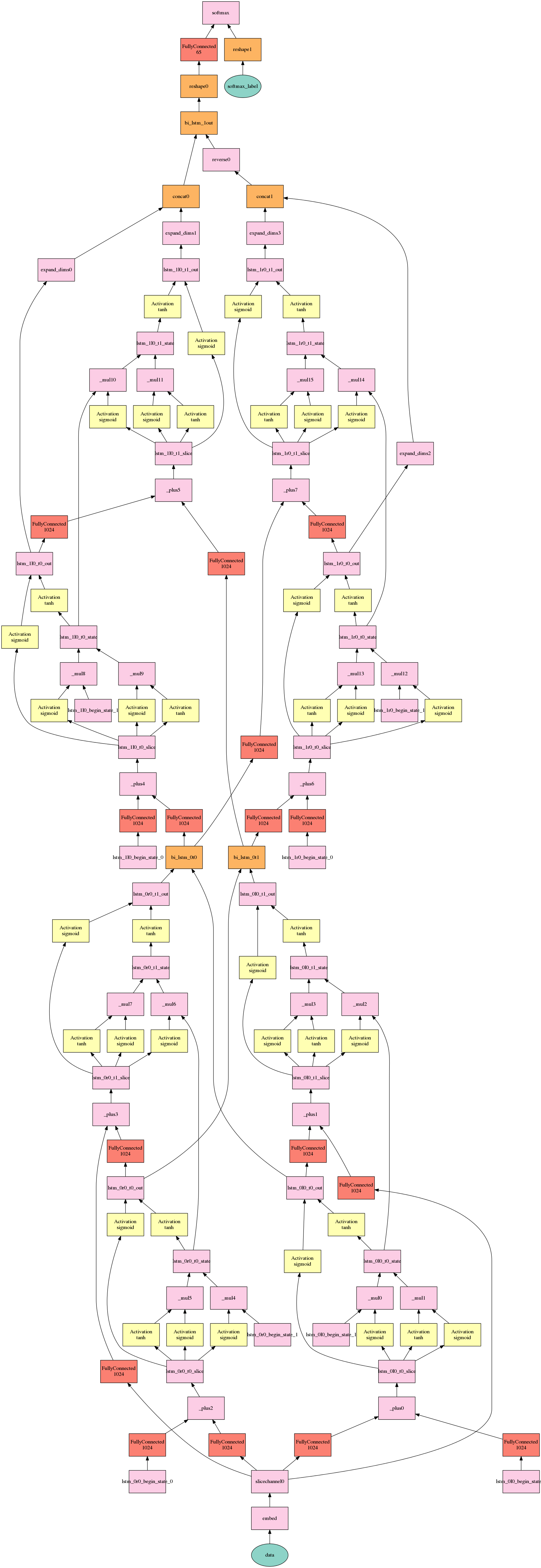The input for the network is a tinyshakespeare sample (about 1mb of Shakespeare works) and the task is to learn the probability distribution of characters in the text to be able to output random Shakespeare like text.
For the text generation I constructed the inference model with the batch size of one and use Math::Random::Discrete to add a bit of randomness into the network inferred character choices.
The sequence size is 60 characters, I seed network with first 60 characters from the tinyshakespeare text, and then ask the network to predict next character, shift the input one character to the left, place the generated character into the last place in the sequence and repeat the process for the sample size.
Below few examples of the generated text.
```WARWICK:
By this, sir, here, take that, what we may belieg,
So, to sue our sons and great persons with thee;
The jriar's mail-shall, to prize our flesh a rose,
Shilling else, which should my constant maid to shameffance,
Even all I beg; would Have us so gentle;
Nothing to be alope.

BARNARDINE:
Then, forb, you would save you.

VOLUMNIA:
Good morrow, night.

Jebus, present us.

ANTONIO:
Even he that ever sink, I'll ries again;
But straight that thou shouldst hear me: farewell,
Boying living sorrow the subject o' the way!

DUKE VINCENTIO:
Kill, your son Bianca, Ratcret's would all.

GONZALO:
He hath soon govern you; rest you call them, I said
Hour too hate I spent in this majesty.

SICINIUS:
'Tis A happy livery,
That I want for peace on your liding in
The govergy drunk! hence!

CAPULET:
High love slain, my meaning.

Shepherd:
Would you beat this Tower? so prois your followers.
O brawling ran, may you remain! I have
left u me ago now to do myself
Above no scales apparel thanks.
BRUTUS:
What is my debating be?
That thou wouldst do incommand till none that
The louding heart with his best once? Holding him!
You shall know by my soul with me like a parent,--
All my living rich some means virtue lie.

YORK:
Now, then, I mused to his chastity.

DUKE OF AUMERLE:
I was my son,
Her officers are past upon my tent.
I'll be park'd these state from the wars to the
cause and thriving , renight, to wive me a
place what vows more than that, which I amouse me death?
A thousand, hang, can you but live?

STANLEY:
Produce it no other, if you command.
Would thy tongue
Bend my bodies?

First Murderer:
No, my mother, where's eish? my man impact
Even in Edward that do disdain, justice,
I'll give this purpose to counterfeit at eye.
Now, you say, sir.

JULIET:
Were Clifford; I be content to wind a change,
While you profess,--you chance,' I shall between sue
As mien unto his own; Sent but this hand,
Your breast dishonours unto my old,
There's proposing time to enjoice their blood: then,
But tell ever such senseless shore, bearing me
Our rogues my life in her mind that slipp'd me
From the king in Rome are question'd king,
And I'll not possible to sleep, the Lords
That done to Rome me spent of virtue.

AUTOLYCUS:
By any wy'll please yourself you can,
How far is ever once again to pardon
Then you stays to speak barren eyes, if once!

Third Servingman:
Harry of any thing on Clarence, shall you go?

GAENENIUS:

ANGELO:
What idle-faced Barnardine! 'tis a villain:
If you, great rest, you shall have drumf, sir.

LUCIO:
What have you our grace hath done to play more
To would rather might be profit the answer,
In war of love and Oxford, dishonour'd hidewh
time and Ten the black morning with usuries,
And seem'd for 'twere now, from the tribunes of me.

ROMEO:
By wholesome great confidence but rushing;
Richard looks to the night to death, but wisdow.
If you'll have some-querb'd and queen in hope:
Things through your worthy Catesby, sir, was much
execution!

```

I've attached 200kb file for your amusement, 20000 char per each of 10 epoch I trained this network for, showing the network getting progressively better, though it's hard to be a good poet if you have just few thousand neurons :-)
Hopefully these examples will look interesting enough to some of you to start experimenting with AI::MXNet :-)
Before signing off I'd like to extend my deepest gratitude to my friend and colleague Robert Stone who kindly provided the source of the calculator and mnist examples for my dissection.I blog about Perl.